# 2.6.2.8.1 Quantitative uncertainty analysis

Page 46 of 59

As outlined in Section 2.6.2.1.2.3, the quantitative uncertainty analysis for the GW AEM is limited to a Monte Carlo sampling of the prior parameter distributions.

Section 2.6.2.8.1.1 summarises the sampled parameter distributions, followed by a discussion of the resulting predictive distributions for the model nodes.

## 2.6.2.8.1.1 Parameter distributions

Figure 16 shows histograms of the 10,000 parameter combinations that are randomly sampled from the parameter distributions summarised in Table 12 and discussed in Section 2.6.2.6.Figure 16 Histograms of posterior parameter distributions

Data: Bioregional Assessment Programme (Dataset 1)

## 2.6.2.8.1.2Predictive distributions

The parameter combinations shown in Figure 16 are evaluated with the GW AEM, and dmax and tmax are computed for the model nodes and for each hydrostratigraphic unit at the model nodes, shown in Figure 10 in Section2.6.2.4. Before discussing in more detail the predicted hydrological change at the model nodes, it is verified if the number of samples – 10,000 – is sufficient. Figure 17 shows the convergence of the 5th, 50th and 95th percentile of drawdown due to additional coal resource development (additional drawdown) for three selected model nodes. For each of those model nodes, it is clear that at least 1000 samples are needed before the quantiles of the ensembles of prediction start to stabilise. From 5000 samples onwards, the quantiles appear not to change anymore. The selected sample size of 10,000 can therefore be considered as sufficient to characterise the median predicted drawdown and the associated 90% confidence interval. Although not shown in this product, during the analysis of the results, it is verified that dmax and tmax converge for all model nodes.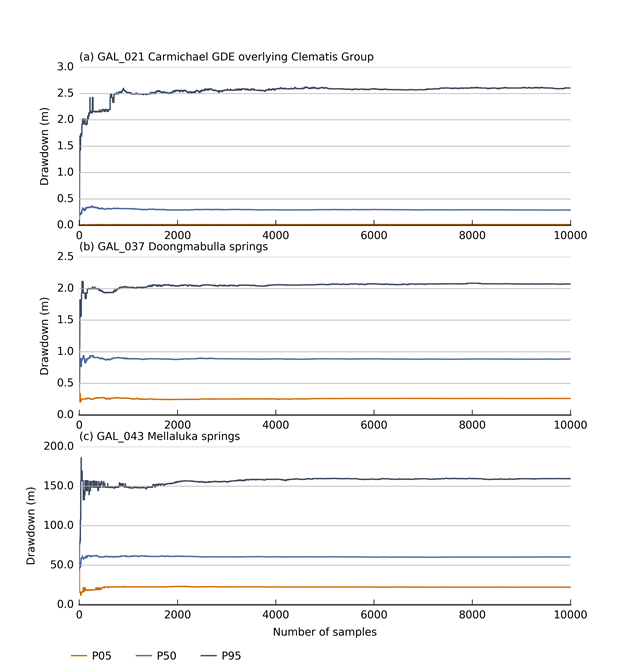Figure 17 Convergence of 5th, 50th and 95th percentile of additional drawdown at selected model nodes of the Galilee subregion

Additional drawdown is dmax, the maximum difference in drawdown between the coal resource development pathway (CRDP) and baseline, due to additional coal resource development.

GDE = groundwater-dependent ecosystem

Data: Bioregional Assessment Programme (Dataset 1)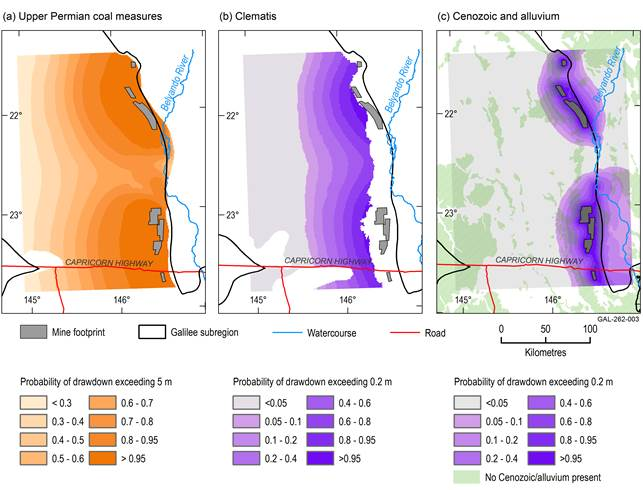Figure 18 Probability of additional drawdown exceeding (a) 5 m in the upper Permian coal measures, (b) 0.2 m in the Clematis Group aquifer and (c) 0.2 m in the Cenozoic and alluvial aquifer

Additional drawdown is dmax, the maximum difference in drawdown between the coal resource development pathway (CRDP) and baseline, due to additional coal resource development.

Data: Bioregional Assessment Programme (Dataset 2)

### Cenozoic and alluvium

Figure 19 shows the median and 90% prediction interval for dmax and tmax at the model nodes associated with the Cenozoic and alluvium hydrostratigraphic unit. Figure 18c shows the probability of dmax exceeding 0.2 m at the model nodes of the GW AEM in the alluvial hydrostratigraphic unit. The 0.2 m threshold is consistent with the threshold defined by QWC (2012) for impacts on springs.

For the majority of the model nodes the median drawdown in alluvium is less than 5 m and maximum change will occur near the end of the simulation period, after the peak coal production period (see Figure 28 in companion product 2.3 for the Galilee subregion (Evans et al., 2018b)). As the mine footprints in the Cenozoic and alluvial aquifer are implemented as a constant drawdown for the entire simulation period, any year of maximum change before the end of the simulation period indicates propagation of the depressurisation in the upper Permian coal measures.

Figure 18 shows that the change is greatest nearer to the mine footprints and decreases with increasing distance from the mining operations. Beyond 25 km from a mine footprint the probability of dmax exceeding 0.2 m is generally less than 5%.Figure 19 (a) additional drawdown (dmax) and (b) year of maximum change (tmax) at the model nodes associated with the Cenozoic and alluvium hydrostratigraphic unit in the Galilee subregion

Additional drawdown is dmax, the maximum difference in drawdown between the coal resource development pathway (CRDP) and baseline, due to additional coal resource development.

Data: Bioregional Assessment Programme (Dataset 1)

### Clematis Group

Figure 20 shows the median and 90% prediction interval of dmax and tmax at the model nodes associated with the Clematis Group hydrostratigraphic unit. Figure 18b shows the probability of dmax exceeding the 0.2 m threshold.

At the model nodes the drawdown is generally less than 2 m and will occur on or after the end of the simulation period. This also means that the additional drawdown is not achieved during the simulation period. From the sensitivity analysis it is clear that simulated drawdown in the Clematis Group aquifer is a propagation of the drawdown in the Cenozoic cover sediments. As the Clematis Group aquifer is simulated as a confined unit, with a specific storage rather than specific yield, the same change in flux will result in a larger drawdown in the Clematis Group aquifer than in the Cenozoic aquifer. This explains the larger spatial extent of the probability contour of more than 5% probability of exceeding 0.2 m drawdown.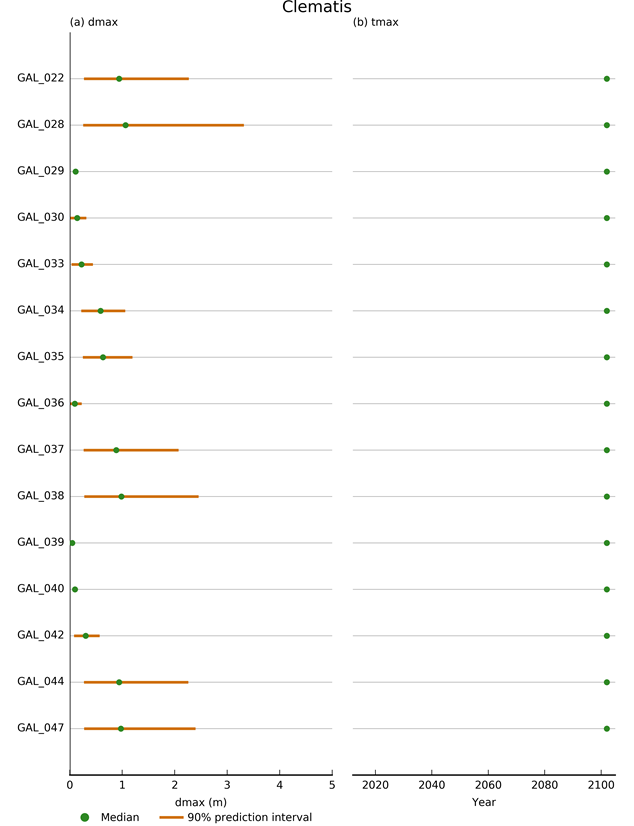Figure 20 (a) additional drawdown (dmax) and (b) year of maximum change (tmax) at the model nodes associated with the Clematis Group hydrostratigraphic unit in the Galilee subregion

Additional drawdown is dmax, the maximum difference in drawdown between the coal resource development pathway (CRDP) and baseline, due to additional coal resource development.

Data: Bioregional Assessment Programme (Dataset 1)

### Upper Permian coal measures

Figure 21 shows the median and 90% prediction interval of the additional drawdown and year of maximum change at the model nodes associated with the upper Permian coal measures hydrostratigraphic unit. Figure 18a shows the probability of dmax exceeding the threshold for consolidated aquifers in Queensland, which is 5 m, at the model nodes in the upper Permian coal measures hydrostratigraphic unit.

Additional drawdowns at the model nodes in the upper Permian coal measures are sizeable with the upper boundary of 90% prediction interval in excess of 100 m for most model nodes. The year of maximum change is mostly realised within the simulation period and close to the peak coal production time.

Figure 18a shows that within the model domain, the probability of exceeding 5 m drawdown is relatively high everywhere. Even at a distance of more than 100 km west of the mine footprints, the probability of exceeding 5 m drawdown is still in excess of 20%.Figure 21 (a) additional drawdown (dmax) and (b) year of maximum change (tmax) at the model nodes associated with the upper Permian coal measures hydrostratigraphic unit in the Galilee subregion

Additional drawdown is dmax, the maximum difference in drawdown between the coal resource development pathway (CRDP) and baseline, due to additional coal resource development.

Data: Bioregional Assessment Programme (Dataset 1)

### Surface water – groundwater flux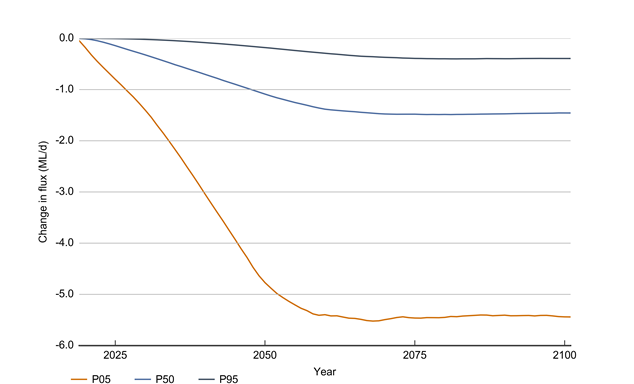Figure 22 5th, 50th and 95th percentile of change in surface water - groundwater flux for the Belyando River

Data: Bioregional Assessment Programme (Dataset 1)

The 5th, 50th and 95th percentile of the change in total surface watergroundwater flux for the Belyando River is shown in Figure 22. The median change in flux at the end of the simulation period is close to 1.5 ML/day, with the 5th percentile around 0.3 ML/day and the 95th percentile close to 5.5 ML/day.

In Section 2.1.5 (surface water – groundwater interaction) in companion product 2.1-2.2 for the Galilee subregion (Evans et al., 2018a), the mean annual baseflow at Belyando Crossing is estimated to be 83 GL/year or 228 ML/day. The 5th, 50th and 95th percentile of change in baseflow due to coal mining therefore corresponds to 0.1%, 0.6% and 2.4% of baseflow at Belyando Crossing, respectively. Companion product 2.6.1 for the Galilee subregion (Karim et al., 2018) provides a more detailed and nuanced discussion on the hydrological change in different river reaches.

The simulated change in surface water – groundwater flux corresponds to about 1% of the pumping rates assigned to the mine footprints (see Table 11 in Section 2.6.2.5). This implies that, as there is no other source of water simulated in the GW AEM, the vast majority of the pumping rate is the depletion of the aquifer storage.

The maximum change is attained close to the time of maximum coal production. The change decreases very slowly after reaching this maximum. In the absence of any other sources of water, the Belyando River is the only source that compensates the cone of depression formed in the upper Permian coal measures, while maintaining the gradient to the constant drawdown at the mine footprints in the Cenozoic hydrostratigraphic unit. The rate of recovery is therefore very likely under estimated.

### Comparison to other groundwater models

The only other regional groundwater model that encompasses all of the modelled developments, is the MODFLOW-USG model developed by HydroSimulations (Turvey et al., 2015). This is a deterministic model in which the pumping rates of the mines are calculated based on the modelled elevation of the coal seam floor (see Section 2.6.2.2). The total cumulative predicted mine pumping rates total 2822 GL, which is about twice the total cumulative mine pumping rate assigned to the analytic element model, which is 1361 GL.

Due to differences in conceptualisation, such as the definition of hydrostratigraphic units and the number of model layers, it is not straightforward to directly compare the predicted drawdowns. An in-depth comparison between the numerical model and analytic element model is therefore not warranted; a fair comparison is only possible when a common benchmark is available. As this is a greenfield site, there is no such common benchmark (e.g. historical drawdown caused by a known pumping rate).

The MODFLOW-USG model does not predict any drawdowns in the regolith layer, which corresponds to the Cenozoic / Alluvial model layer in the analytic element model. The MODFLOW-USG model does not simulate direct mine drainage in the regolith layer, which explains the discrepancy in model results.

The simulated drawdown in the Clematis Group, layer 8 in the MODFLOW-USG model, is less than 5 m. At the model nodes in the Clematis Group, the analytic element model predicted drawdowns do not exceed 4 m, although at several model nodes the median drawdown is close to 1 m. The contour of 50% probability of exceeding 0.2 m in Figure 18b corresponds broadly to the 1 m drawdown contour in layer 8 at 2090 (Turvey et al., 2015, p. 220).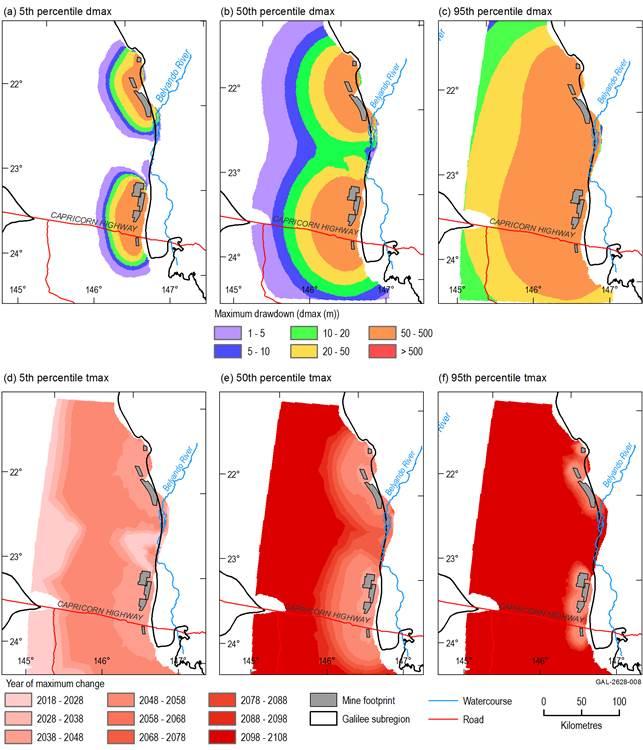Figure 23 Contour maps of the 5th, 50th and 95th percentile of additional drawdown (top row) and year of maximum change (bottom row) in the upper Permian coal measures

Additional drawdown is dmax, the maximum difference in drawdown between the coal resource development pathway (CRDP) and baseline, due to additional coal resource development.

tmax = year of maximum change

Data: Bioregional Assessment Programme (Dataset 2)

Figure 23 shows the 5th, 50th and 95th percentile of additional drawdown and year of maximum change in the upper Permian coal measures simulated with the analytic element model. As such, these results are not directly comparable to the results presented in Turvey et al. (2015) since in the numerical model, the upper Permian coal measures are divided into several layers and the results are presented as maps of drawdown at specific points in time, rather than the additional drawdown and year of maximum change. The drawdown map for layer 12 in 2058 (Turvey et al., 2015, p. 264) is reproduced in Figure 24. This shows the drawdown in the Betts Creek beds formation seam E. The map for 2058 is chosen as this time slice appears to have the maximum extent of drawdown.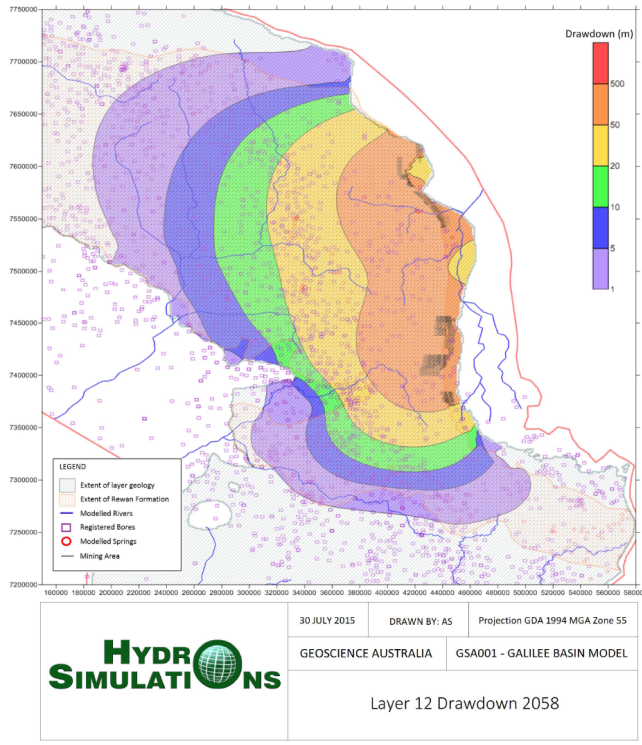Figure 24 Drawdown in layer 12 (Betts Creek beds formation seam E) in 2058

Source: Turvey et al. (2015)

The drawdown contours depicted in Figure 24 are very similar to the contours shown in Figure 23c, the 95th percentile of additional drawdown. This is not unexpected as the pumping rates in the MODFLOW model are almost twice those applied to the analytic element model, and the calibrated horizontal hydraulic conductivities for the upper Permian coal measures in the MODFLOW model, which range from 0.13 to 2.5 m/day, are close to the 95th percentile of the parameter distribution for the horizontal hydraulic conductivity of the upper Permian coal measures (Figure 23).

A direct comparison between the predicted changes in baseflow between both models is not straightforward due to the differences in the conceptualisation and implementation of surface water – groundwater interaction. Table 5-2 in Turvey et al. (2015) lists a 0.2% reduction in baseflow in the Belyando River at the model edge. This is the same order of magnitude of baseflow reduction percentage as simulated with the GW AEM. Note that the hydrological change of the streamflow-related hydrological response variables is reported in companion product 2.6.1 for the Galilee subregion (Karim et al., 2018). In the surface water modelling the change in surface water – groundwater interaction flux from the GW AEM is combined with the reduction in runoff associated with coal mining.

Last updated:
17 January 2019